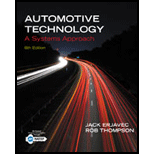Chapter 3, Problem 4RQAutomotive Technology: A Systems A...

6th Edition
Jack Erjavec + 1 other
ISBN: 9781133612315

Solutions

Chapter
SectionAutomotive Technology: A Systems A...

6th Edition
Jack Erjavec + 1 other
ISBN: 9781133612315
Textbook Problem

Describe five different forms of energy.

To determine

Different forms of energy.

Explanation

Given Information:

Different type of energy

Kinetic energy:- Energy caused due to motion is called Kinetic energy.

Mathematically representation

E=12mv 2

Where,

E = Kinetic energy

m= mass of body or object

v= velocity of body or object

Example: Any moving object.

Potential Energy:-Potential energy is the energy of an object's position.

Mathematically representation

E= mgh

Where,

E= Potential energy

M= mass of object

g= acceleration

h= height from datum of object

Example: An object at a height

Still sussing out bartleby?

Check out a sample textbook solution.

See a sample solution

The Solution to Your Study Problems

Bartleby provides explanations to thousands of textbook problems written by our experts, many with advanced degrees!

Get Started

What two angles can be laid out with the combination square?

Precision Machining Technology (MindTap Course List)

What is a DBMS, and what are its functions?

Database Systems: Design, Implementation, & Management

What is cloud computing? How is it used?

Fundamentals of Information Systems

Calculate the volume of material used in making 100 ft of 4 in. type M copper tubing.

Engineering Fundamentals: An Introduction to Engineering (MindTap Course List)